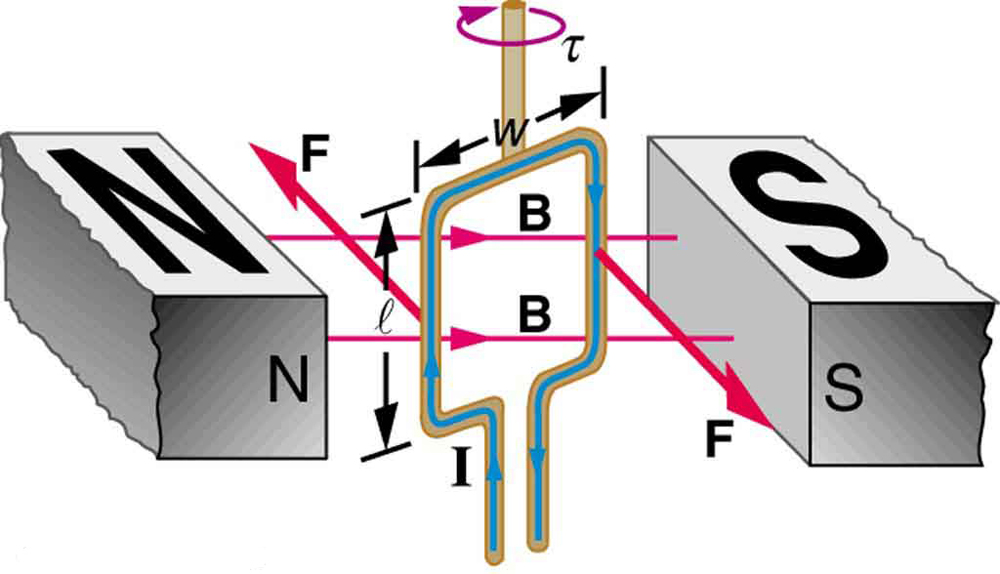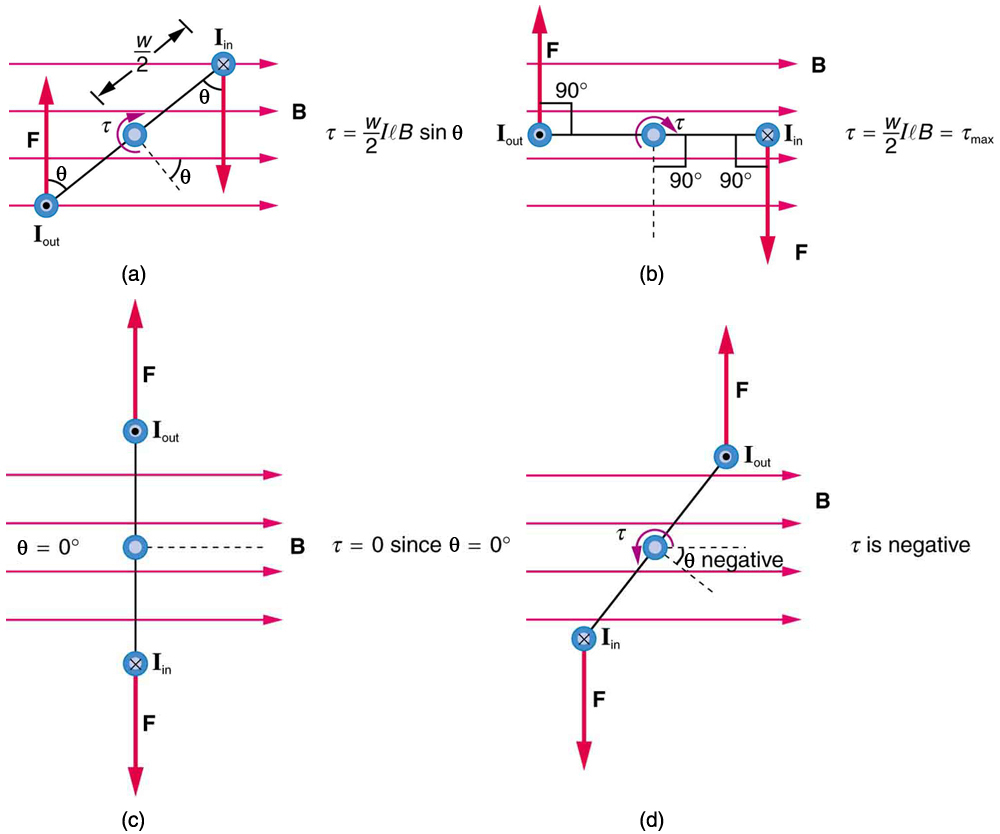# 22.8 Torque on a current loop: motors and meters

 Page 1 / 4
• Describe how motors and meters work in terms of torque on a current loop.
• Calculate the torque on a current-carrying loop in a magnetic field.

Motors are the most common application of magnetic force on current-carrying wires. Motors have loops of wire in a magnetic field. When current is passed through the loops, the magnetic field exerts torque on the loops, which rotates a shaft. Electrical energy is converted to mechanical work in the process. (See [link] .)Torque on a current loop. A current-carrying loop of wire attached to a vertically rotating shaft feels magnetic forces that produce a clockwise torque as viewed from above.

Let us examine the force on each segment of the loop in [link] to find the torques produced about the axis of the vertical shaft. (This will lead to a useful equation for the torque on the loop.) We take the magnetic field to be uniform over the rectangular loop, which has width $w$ and height $l$ . First, we note that the forces on the top and bottom segments are vertical and, therefore, parallel to the shaft, producing no torque. Those vertical forces are equal in magnitude and opposite in direction, so that they also produce no net force on the loop. [link] shows views of the loop from above. Torque is defined as $\tau =\text{rF}\phantom{\rule{0.25em}{0ex}}\text{sin}\phantom{\rule{0.25em}{0ex}}\theta$ , where $F$ is the force, $r$ is the distance from the pivot that the force is applied, and $\theta$ is the angle between $r$ and $F$ . As seen in [link] (a), right hand rule 1 gives the forces on the sides to be equal in magnitude and opposite in direction, so that the net force is again zero. However, each force produces a clockwise torque. Since $r=w/2$ , the torque on each vertical segment is $\left(w/2\right)F\phantom{\rule{0.25em}{0ex}}\text{sin}\phantom{\rule{0.25em}{0ex}}\theta$ , and the two add to give a total torque.

$\tau =\frac{w}{2}F\phantom{\rule{0.25em}{0ex}}\text{sin}\phantom{\rule{0.25em}{0ex}}\theta +\frac{w}{2}F\phantom{\rule{0.25em}{0ex}}\text{sin}\phantom{\rule{0.25em}{0ex}}\theta =\text{wF}\phantom{\rule{0.25em}{0ex}}\text{sin}\phantom{\rule{0.25em}{0ex}}\theta$Top views of a current-carrying loop in a magnetic field. (a) The equation for torque is derived using this view. Note that the perpendicular to the loop makes an angle θ size 12{θ} {} with the field that is the same as the angle between w / 2 size 12{w/2} {} and F size 12{F} {} . (b) The maximum torque occurs when θ size 12{θ} {} is a right angle and sin θ = 1 size 12{"sin"θ=1} {} . (c) Zero (minimum) torque occurs when θ size 12{θ} {} is zero and sin θ = 0 . (d) The torque reverses once the loop rotates past θ = 0 .

Now, each vertical segment has a length $l$ that is perpendicular to $B$ , so that the force on each is $F=\text{IlB}$ . Entering $F$ into the expression for torque yields

$\tau =\text{wIlB}\phantom{\rule{0.25em}{0ex}}\text{sin}\phantom{\rule{0.25em}{0ex}}\theta .$

If we have a multiple loop of $N$ turns, we get $N$ times the torque of one loop. Finally, note that the area of the loop is $A=\text{wl}$ ; the expression for the torque becomes

$\tau =\text{NIAB}\phantom{\rule{0.25em}{0ex}}\text{sin}\phantom{\rule{0.25em}{0ex}}\theta .$

This is the torque on a current-carrying loop in a uniform magnetic field. This equation can be shown to be valid for a loop of any shape. The loop carries a current $I$ , has $N$ turns, each of area $A$ , and the perpendicular to the loop makes an angle $\theta$ with the field $B$ . The net force on the loop is zero.

## Calculating torque on a current-carrying loop in a strong magnetic field

Find the maximum torque on a 100-turn square loop of a wire of 10.0 cm on a side that carries 15.0 A of current in a 2.00-T field.

Strategy

Torque on the loop can be found using $\tau =\text{NIAB}\phantom{\rule{0.25em}{0ex}}\text{sin}\phantom{\rule{0.25em}{0ex}}\theta$ . Maximum torque occurs when $\theta =\text{90º}$ and $\text{sin}\phantom{\rule{0.25em}{0ex}}\theta =1$ .

Solution

For $\text{sin}\phantom{\rule{0.25em}{0ex}}\theta =1$ , the maximum torque is

${\tau }_{\text{max}}=\text{NIAB}.$

Entering known values yields

$\begin{array}{lll}{\tau }_{\text{max}}& =& \left(\text{100}\right)\left(\text{15.0 A}\right)\left(\text{0.100}\phantom{\rule{0.25em}{0ex}}{\text{m}}^{2}\right)\left(2\text{.}\text{00 T}\right)\\ & =& \text{30.0 N}\cdot m.\end{array}$

Discussion

This torque is large enough to be useful in a motor.

What is the difference between a principle and a law
the law is universally proved. The principal depends on certain conditions.
Dr
it states that mass of an element deposited during electrolysis is directly proportional to the quantity of electricity discharge
Olamide
what does the speedometer of a car measure ?
Car speedometer measures the rate of change of distance per unit time.
Moses
describe how a Michelson interferometer can be used to measure the index of refraction of a gas (including air)
using the law of reflection explain how powder takes the shine off a person's nose. what is the name of the optical effect?
WILLIAM
is higher resolution of microscope using red or blue light?.explain
WILLIAM
what is dimensional consistent
Mohammed
In engineering and science, dimensional analysis is the analysis of the relationships between different physical quantities by identifying their base quantities and units of measure and tracking these dimensions as calculations or comparisons are performed
syed
can sound wave in air be polarized?
Unlike transverse waves such as electromagnetic waves, longitudinal waves such as sound waves cannot be polarized. ... Since sound waves vibrate along their direction of propagation, they cannot be polarized
Astronomy
A proton moves at 7.50×107m/s perpendicular to a magnetic field. The field causes the proton to travel in a circular path of radius 0.800 m. What is the field strength?
derived dimenionsal formula
what is the difference between mass and weight
assume that a boy was born when his father was eighteen years.if the boy is thirteen years old now, how is his father in
Isru
31yrs
Olamide
what is airflow
derivative of first differential equation
why static friction is greater than Kinetic friction
draw magnetic field pattern for two wire carrying current in the same direction
An American traveler in New Zealand carries a transformer to convert New Zealand’s standard 240 V to 120 V so that she can use some small appliances on her trip.
What is the ratio of turns in the primary and secondary coils of her transformer?
nkombo
what is energy
Yusuf
How electric lines and equipotential surface are mutually perpendicular?
The potential difference between any two points on the surface is zero that implies È.Ŕ=0, Where R is the distance between two different points &E= Electric field intensity. From which we have cos þ =0, where þ is the angle between the directions of field and distance line, as E andR are zero. Thus
sorry..E and R are non zero...

#### Get Jobilize Job Search Mobile App in your pocket Now!ByBy Brooke DelaneyBy OpenStaxBy Madison ChristianBy Brooke DelaneyBy Michael NelsonByBy Carly AllenBy Jonathan LongBy Angela JanuaryBy OpenStax